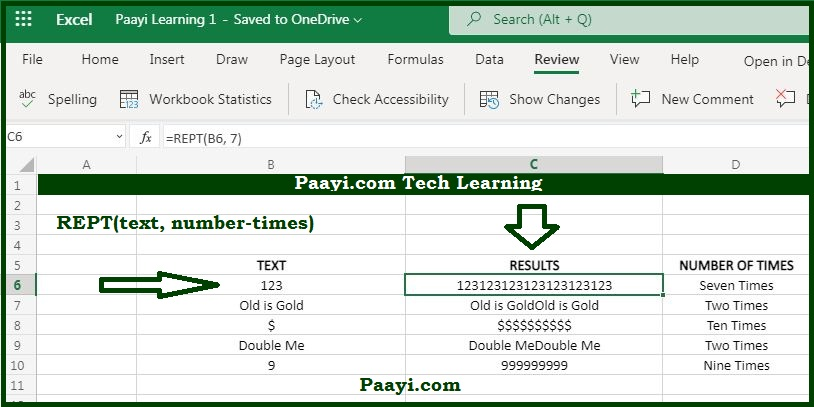# Learn How to use Microsoft Excel REPT Function

Written by | 0 Comments | 830 Views

In this article, you will learn how to use the Microsoft Excel REPT function and its prime function in Microsoft Excel. You will also get to know the Microsoft Excel REPT function return value and syntax with the help of some examples.

## Microsoft Excel REPT Function

The Microsoft Excel REPT function is used to repeat the text as specified. That means with the help of the REPT function; you can repeat the characters for the given number of times. Let's understand this by the example provided, the formula:

= REPT("A", 5) will return the value - "AAAAA."

### REPT Function Return Value

It will repeat the specified text for a given time.

### REPT Function Syntax

= REPT(text, number-times)

Where the arguments:

• text: The text you want to repeat.
• number-times: The number of times you want to repeat the specified text string.

## How to Use the Microsoft Excel REPT Function?As we know, with the help of the REPT function, you can able to repeat the specified times for a given number of times. Let us understand this by the examples provided below:

Example 1: = REPT("A" 5) - It will return the value "AAAAA."

Example 2: If you want to repeat the text in B5, by the count in C5, you can use this formula:

= REPT(B5, C5) - This will return the text in the B5 by the count provided in the C5.